Next: Subdivision curves and surfaces Up: No Title Previous: B-splines

Subsections

# Rational B-splines

Rational B-splines have all of the properties of non-rational B-splines plus the following two useful features:
• They produce the correct results under projective transformations (while non-rational B-splines only produce the correct results under affine transformations).
• They can be used to represent lines, conics, non-rational B-splines; and, when generalised to patches, can represents planes, quadrics, and tori.

The antonym of rational is non-rational. Non-rational B-splines are a special case of rational B-splines, just as uniform B-splines are a special case of non-uniform B-splines. Thus, non-uniform rational B-splines encompass almost every other possible 3D shape definition. Non-uniform rational B-spline is a bit of a mouthful and so it is generally abbreviated to NURBS.

We have already learnt all about the the B-spline bit of NURBS and about the non-uniform bit. So now all we need to know is the meaning of the rational bit and we will fully(?) understand NURBS.

Rational B-splines are defined simply by applying the B-spline equation (Equation 87) to homogeneous coordinates, rather than normal 3D coordinates. We discussed homogeneous coordinates in the IB course. You will remember that these are 4D coordinates where the transformation from 4D to 3D is: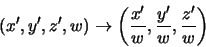(94)

Last year we said that the inverse transform was: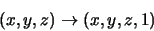(95)

This year we are going to be more cunning and say that: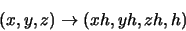(96)

Thus our 3D control point,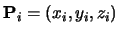, becomes the homogeneous control point,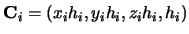.

A NURBS curve is thus defined as:(97)

Compare Equation 97 with Equation 87 to see just how easy this is!

We now want to see what a NURBS curve looks like in normal 3D coordinates, so we need to apply Equation 94 to Equation 97. In order to better explain what is going on, we first write Equation 97 in terms of its individual components. Equation 97 is equivalent to:

 x'(t) =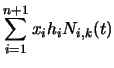(98) y'(t) =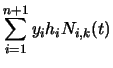(99) z'(t) =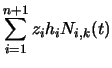(100) h(t) =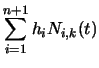(101)

Equation 94 tells us that, in 3D:
 x(t) = x'(t)/h(t) (102) y(t) = y'(t)/h(t) (103) z(t) = z'(t)/h(t) (104)

Thus the 4D to 3D conversion gives us the curve in 3D: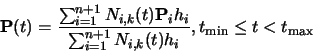(105)

This looks a lot more fierce than Equation 97, but is simply the same thing written a different way.

So now, we need to define an additional parameter, hi, for each control point,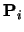. The default is to set. This results in the denominator of Equation 105 becoming one, and the NURBS equation (Equation 105) therefore reducing to the non-rational B-spline equation (Equation 87).

Increasing hi pulls the curve closer to point. Decreasing hi pushes the curve farther from point. Setting hi=0 means thathas no effect on the curve at all.

## An example: a circle defined by NURBS

A non-rational B-spline or a Bezier curve cannot exactly represent a circle. An interesting exercise is to place a cubic Bezier curve's end points at (0,1) and (1,0), with the other control points at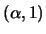and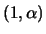. Now see how close this quarter circle'' comes to the real quarter circle defined by x2 + y2 = 1, i.e. what is the value of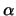for which the Bezier curve most closely matches the quarter circle.

NURBS can be used to represent circles, and all of the other conics. NURBS surfaces can be used to represent quadric surfaces. As an example, let us consider one way in which NURBS can be used to describe a true circle. Rogers and Adams cover this on pages 371-375.

Construct eight control points in a square. Let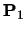,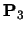,, and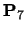be the vertices of the square. Let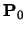,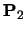,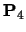, and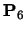be the midpoints of the respective sides, so that the vertices are numbered sequentially as you proceed around the square. Finally, you need a ninth point to join up the curve, so let.

Use a quadratic B-spline basis function with the knot vector
[0,0,0,1,1,2,2,3,3,4,4,4]. This means that the curve will pass through,,,and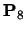, and allows us to essentially treat each quarter of the circle independently.

We finally need to specify the homogeneous co-ordinates. As a circle is symmetrical is should be obvious that that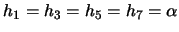and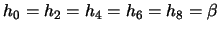. As we would like the curve to pass through the even numbered points we know that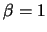. All we therefore need to determine is, the value of the odd numbered homogeneous co-ordinates.

If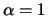then the NURBS curve will bulge out more than a circle. If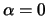, it will bow in. This gives us limits on the value of. To find the exact value we take one quarter of the NURBS curve definition: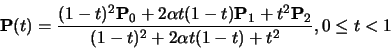(106)

Assume now that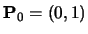,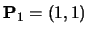, and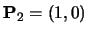. Insert Equation 106 into the equation for the unit circle ( x(t)2+y(t)2=1). The resulting equation is: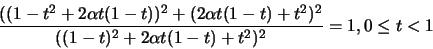(107)

Now solve this for. Equation 107 is essentially:(108)

From this we can conclude that we require aN=aD, bN=bD, cN=cD, dN=dD, and eN=eD. The first three all solve to give the result that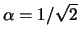, while the last two cancel out totally to give the tautology 0=0. Thus. If you are in a hurry to solve this, and you can remember that each of the first three equations gives the same answer then you need simply extract the coefficients of t4 from Equation 107 and solve. It is even easier if you remember the magic number,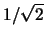.

## Sweeping with NURBS

A NURBS surface is defined as the same two-dimensional extension to NURBS curves described in Equation 93, though obviously carried out in homogeneous co-ordinates. You can define sweeps using NURBS curves by using one NURBS curve as the sweep path, and another NURBS curve as the cross-section. You take the tensor product of the two curves. This will be described more fully in the lectures and is covered in Rogers & Adams, pages 445-456, 465-477.Next: Subdivision curves and surfaces Up: No Title Previous: B-splines
Neil Dodgson
2000-09-25